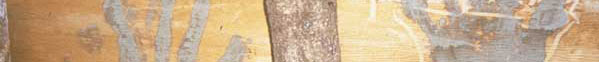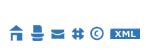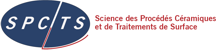Home > Computation engine# Computation engine

## a) thermodynamic properties

by minimization of the free enthalpy by the way Lagrange's multiplicands and the steepest descent algorithm as describe by White et Dantzig. This method known as method of RAND allows the resolution of very complex systems. The Lagrange's multiplicands must be identified to chemical potentials of elements, then the relative precision for the moles number of minor species is the same as for major species.

## b) transport properties

are based on the resolution of Boltzmann's integro-differential equation using the method of Chapman and Enskog. The calculation of the electrical conductivity is performed to the third approximation, as recommended by Devoto. The thermal conductivity is determined as the sum of four components : the heavy species translational thermal conductivity, the electron translational thermal conductivity, the internal thermal conductivity, all developed at the second approximation, and the reaction conductivity calculated according to the theory of Butler and Brokaw, at the first approximation. The viscosity, which is that of heavy species, is calculated to the first approximation. The interaction potentials used were found in the classical references.

## c) the electron temperature

in a gas under an electric field.

## OTHERS THERMODYNAMIC PROPERTIES COMPUTATION

The computation is based on the research of the composition which minimize the free enthalpy (also named Gibb's enthalpy) G(T,P) of the multiphase system.

Enthalpy H(T,P), internal energy E(T,V) and entropy S(T,P) of the system are immediate, ant the numerical derivation gives:

 Cp = dH/dT Cv = dE/dT γ = Cp/Cv Γ = (dH/dE)S

the sound celerity a in gas phase is : a2 = ΓnRT/M

In the equations above: n is the moles number, M is the molar weigh, T is temperature, P is pressure, V is volume, R is perfect gas constant, Cp is specific heath at constant pressure, Cv is specific heat at constant volume, G is isentropic coefficient.

Computations can be made under conditions:

• isotherm (constant temperature) or adiabatic (without heat exchange, here enthalpy or internal energy constant),
• isobars (constant pressure) or isochors (constant volume).

Also system can be frozen with forbidden reaction (e.g. for evaluation of energy balance).T&TWinner